/

### The logistic distribution

The logistic distribution has a similar shape to the normal distribution but has heavier tails.

 Distribution name Logistic distribution Common notation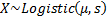Parameters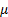= location parameter= scale parameter (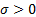) Domain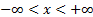Probability density function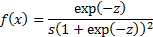where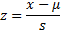Cumulative distribution function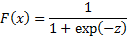MeanVariance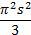Skewness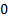(Excess) kurtosis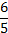Characteristic function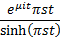Other comments The logistic distribution is sometimes called the sech-square(d) distribution because its pdf can also be expressed in terms of the hyperbolic secan function: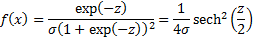It should not then be confused with the hyperbolic secant distribution.

Nematrian web functions

Functions relating to the above distribution may be accessed via the Nematrian web function library by using a DistributionName of “logistic”. For details of other supported probability distributions see here.